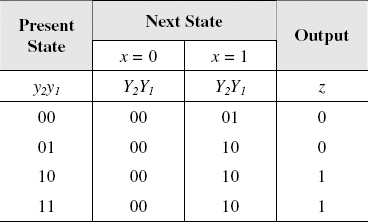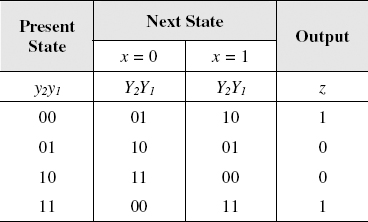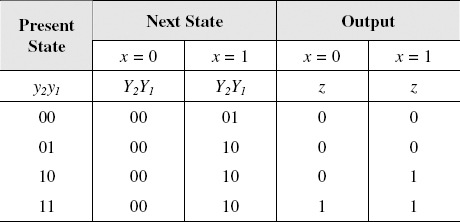## PROBLEMS

9.1 What is a finite-state machine?

9.2 List the procedural steps for finite-state machine design.

9.3 What is a Mealy machine?

9.4 What is a Moore machine, and how does it differ from a Mealy machine?

9.5 Using D flip-flops, design a logic circuit for the finite-state machine described by the state assigned table in Figure P9.5.Figure P9.5

9.6 Using D flip-flops, design a logic circuit for the finite-state machine described by the state assigned table in Figure P9.6.Figure P9.6

9.7 Using D flip-flops, design a logic circuit for the finite-state machine described by the state assigned table in Figure P9.7.

9.8 Using D flip-flops, design a logic circuit for the finite-state machine described by the state assigned table in Figure P9.8.

9.9 Using D flip-flops, design a logic circuit for the finite-state machine described by the state assigned table in Figure P9.9.Figure P9.7Figure P9.8Figure P9.9

9.10 Using D flip-flops, design a logic circuit for the finite-state machine ...

Get Introduction to Digital Systems: Modeling, Synthesis, and Simulation Using VHDL now with the O’Reilly learning platform.

O’Reilly members experience live online training, plus books, videos, and digital content from nearly 200 publishers.